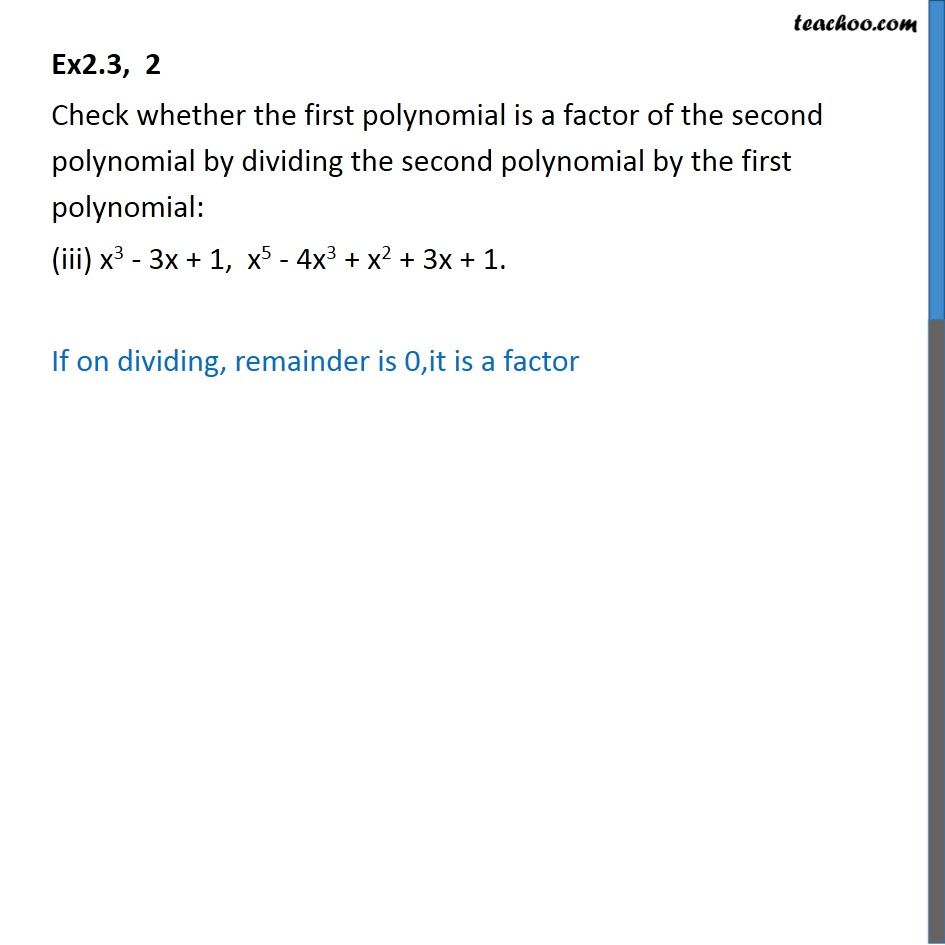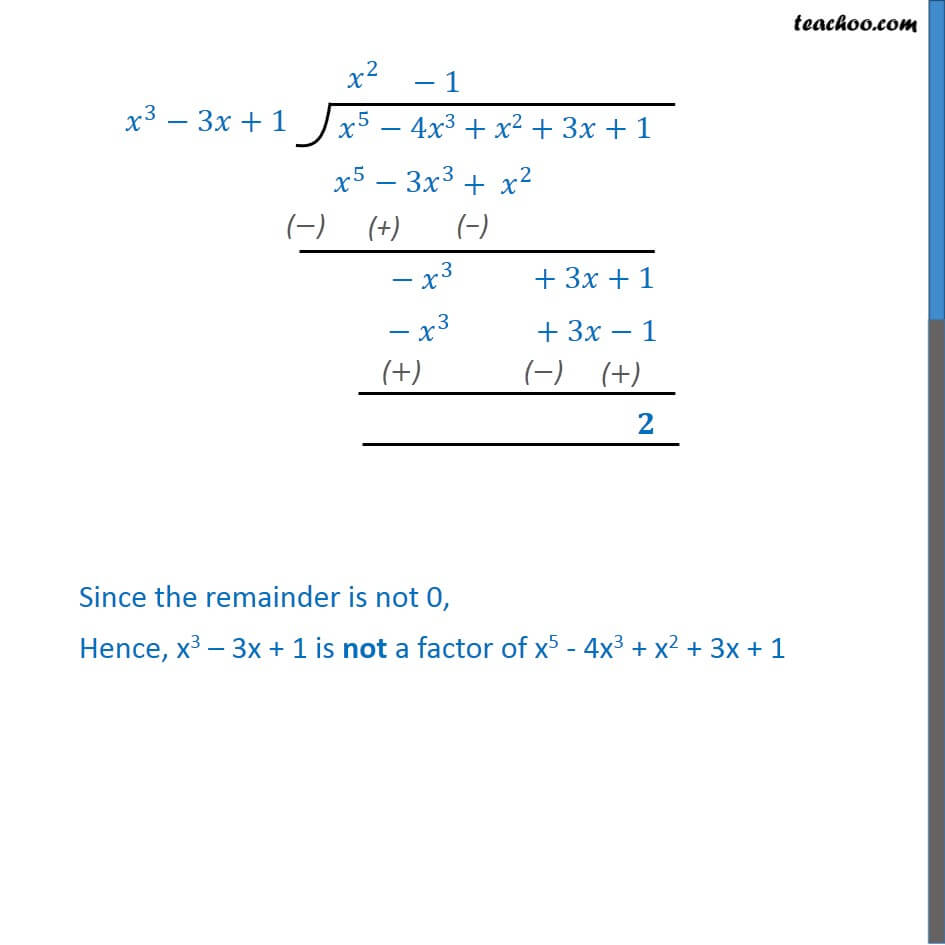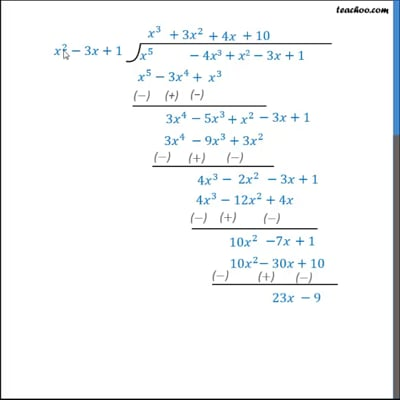Ex 2.3

Chapter 2 Class 10 Polynomials (Term 1)
Serial order wiseThis video is only available for Teachoo black users

### Transcript

Ex2.3, 2 Check whether the first polynomial is a factor of the second polynomial by dividing the second polynomial by the first polynomial: (iii) x3 - 3x + 1, x5 - 4x3 + x2 + 3x + 1. If on dividing, remainder is 0,it is a factor Since the remainder is not 0, Hence, x3 3x + 1 is not a factor of x5 - 4x3 + x2 + 3x + 1